# Monthly Archives: November 2017

## Digging Deeper into … Money (all classes)

Category : Uncategorized

For practical suggestions for families, and helpful links to digital resources, to support children learning about the topic of money, please check out the following post: Dear Family, your Operation Maths Guide to Money

Similar to the strand unit of time, money is an integral element of our daily lives and therefore an essential, “need-to-know” topic in primary maths, particularly for those children with special educational needs or learning difficulties. And, while it is not as abstract as the topic of time, it still can be a concept with which many children struggle. Consider the nature of money itself:

• It comes in different shapes and sizes, and in metal and paper forms (i.e. coins and notes) each of which has its own value.
• The sizes of the coins and notes are not proportional to their value i.e. a 20c coin is not twice as big as a 10c coin; a €100 is not ten times the size of the €10. Therefore, while money can be used as a base-ten material, unlike the base-ten blocks, it is not proportional.
• Money can be expressed as € or c, but not as both. And when using the € sign it precedes the numbers (even though it is verbalised as “six euro” as opposed to “euro six”), where as the c sign comes after the numeral.
• Countries often use different currencies; this can lead to confusion when children presume that dollars and pounds are used in this country, because they hear this terminology regularly from imported TV programmes.
• When changing currencies you cannot do a straight swap i.e. €1 doesn’t equal £1 or \$1; the new value must be calculated using an exchange rate, which also varies.
• More and more, transactions are becoming cashless, as people use credit and debit cards more than ever before. Thus, children are missing out on essential opportunities to handle cash, or see it being handled in real-life situations. The increased use of plastic and contactless payments also limits the opportunities for people to total mentally, calculate change etc.

### Elicit prior knowledge & concrete exploration

At every class level, it is always a good idea to elicit the children’s prior knowledge, which can be very varied, depending on the experiences they’ve had with money. Even some simple revision questions can be very revealing, such as these:

• ‘What currency/money do we use in Ireland?’
• ‘Do you know of any other countries that use the euro?’
• ‘Can you name the coin/note with the least value? And the next? And the next?’ etc

Even in a senior class, the answers to the last question can often be ‘1c, 2c, 3c, 4c…’ as the children forget or don’t realise that there is not a single coin for each value. In keeping with the CPA approach of Operation Maths,  these type of questions should be followed with opportunities to explore and examine the actual notes and coins, and the similarities and differences between them. And, if there is not enough real or replica money, the Sorting eManipulative, accessible via edcolearning.ie, can be a very useful way to display the coins and notes (see below).Do you notice any pattern? Many children, and even some adults, don’t recognise that our euro money follows a ‘1, 2, 5’ pattern i.e. every note or coin has either 1, 2 or 5 as its most significant digit (look at the columns above). Once the children recognise this, they are less likely to suggest using a ‘3c coin’ or a ‘7c coin’ etc to make a value. To improve their familiarity with the coins, even children in junior infants could use them for pre-number sorting purposes, eg using coins for sorting by size, colour, shape etc. They don’t need to be restricted to just coins up to 5c (the traditional limit for junior infants), as the focus is not on number. Play activities based on money e.g. the shop, post office, restaurant etc should also be encouraged, particularly as the basis for Aistear themes.

### Exploring the value of the coins

Recognising the value of each individual coin is one thing, recognising that one coin can be exchanged for a number of coins of equal value is very different. This is why it can be very useful to represent the value of the coins concretely. This can be done by attaching coins to large squared card and/or ten and five frames. Grid paper with 2cm squares is perfect for this. Just print out/photocopy onto white or coloured paper or light card and then cut out into sections that relate to five and ten frames (see image below):

• 1c, 2c, 5c on to strips of 1, 2 or 5 squares respectively
• 10c onto a 2×5 section i.e. ten frame
• 20c onto a 4×5 section, with a bold line through centre to show each ten
• 50c onto a 10×5 section, with 4 bold lines to show each ten

This reinforces the benchmarks of five and ten, while building on the children’s ability to subitise (recognise at a glance) these quantities.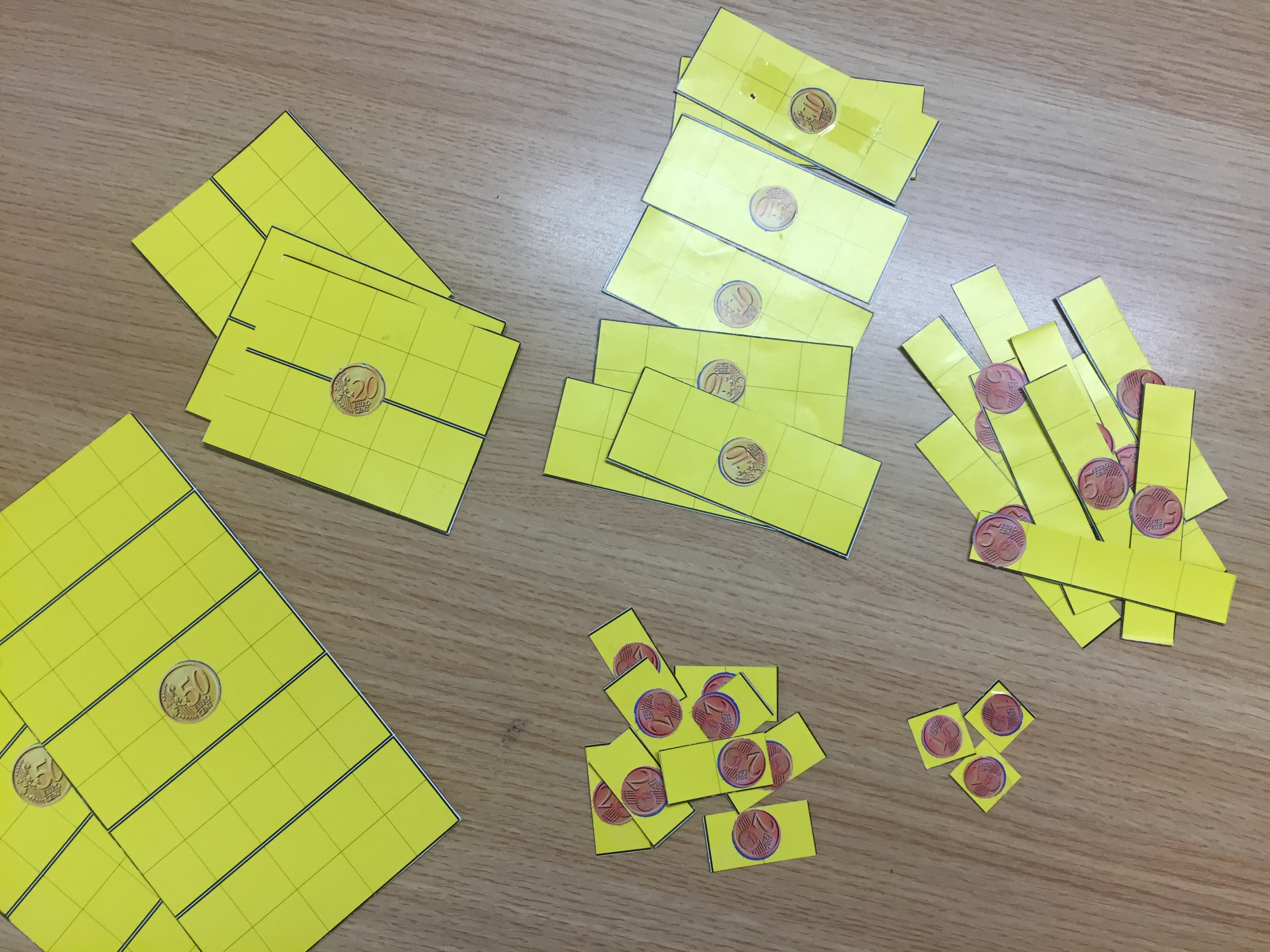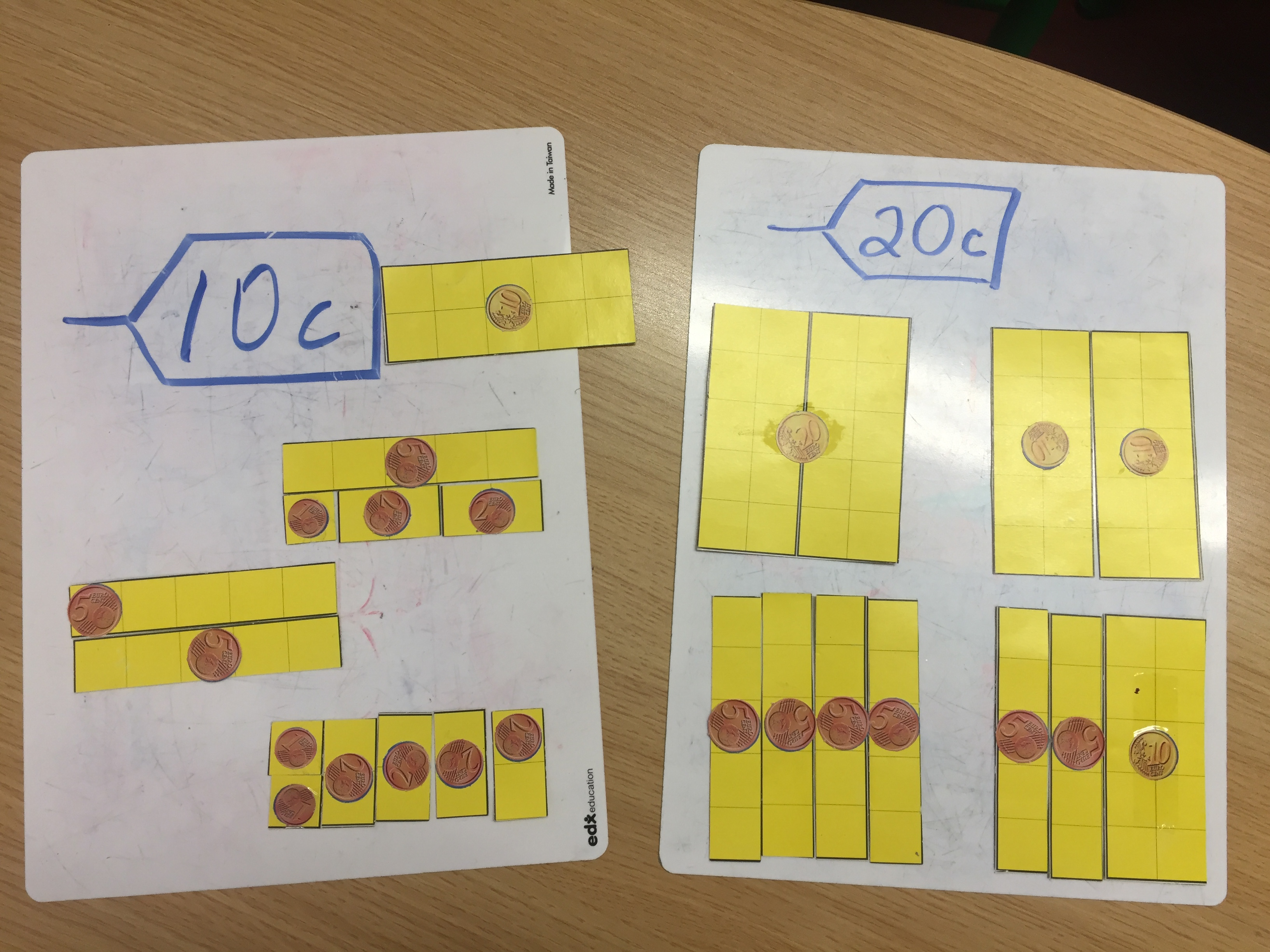These materials can then be used for exchanging activities, where the children identify different ways to make various values, i.e “same value, different appearance”,  e.g. what coins could we use instead of 2c, 5c, 10c, 20c, 50c, etc. When the children are comfortable making these values they should then make values that are not equivalent to a single coin e.g. 6c, 13c, 23c etc. Ultimately, it is hoped that the children will be able to visualise the value of the coins without needing the visual supports shown above.

### Mental calculations with money

Despite the face that our society is becoming increasingly cashless, mental calculations with money should still be emphasised and, in particular, the strategy of making change. Officially referred to a complementary addition, where you add on to subtract, it has also been known as “shopkeepers arithmetic” given its application in those situations. It is also one of the specific subtraction strategies dealt with in Number Talks, where it is referred to as Adding up (all of the other Number Talks strategies are also relevant to calculations involving money, but this one is worthy of a special mention).  Complementary addition is also one of the strategies used in Operation Maths, where it is shown using the visual strategy of an empty number line (see below).### Visual Strategies for Problem Solving

A key element of Operation Maths is the use of three specific visual strategies to support the development of problem solving skills. These are empty number lines (as shown above), bar models and T-charts. T-charts are particularly useful to solve problems based on the unitary method, as shown below.Bar models can be very useful to model addition and subtraction problems e.g. where the whole amount is known and a part is missing or where the parts are known and the whole is missing. The type of models shown below are referred to a part-whole models.### Other tips and suggestions for teaching money

• Emphasise that money is based on the euro. Cent coins are merely fractions of that unit; euro coins and notes are multiples of that unit. In this way money can also be used to help teach other strand units, including place value, operations and decimals (see examples below).
•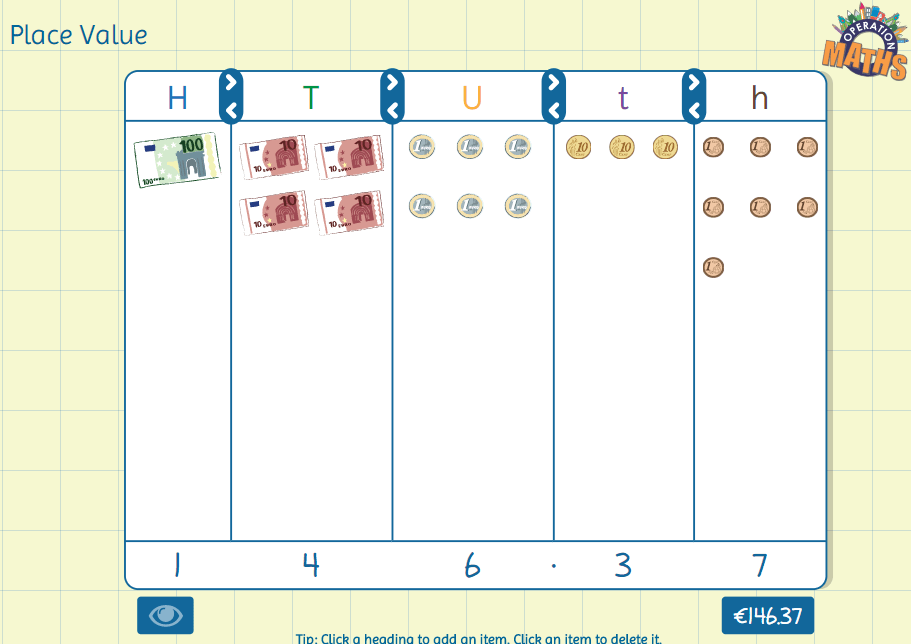Using money to represent place value with whole numbers and decimalsUsing money to model 2-digit division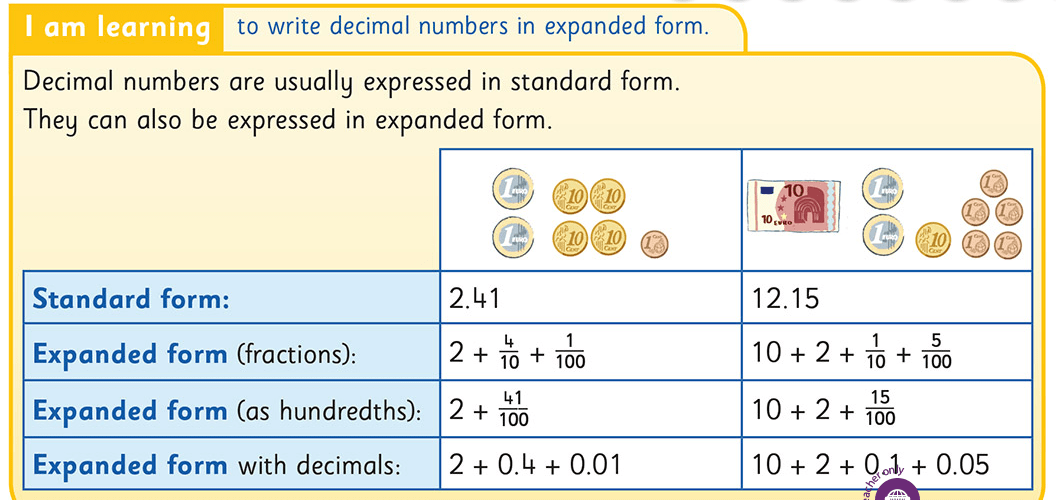Using money to model decimals to hundredths

• Emphasise the importance of using an efficient estimation strategy when calculating with money:
• front-end estimation: where you only consider the most significant digit i.e. think of €26.95 as €20
• rounding: where you round to the nearest unit, ten etc, i.e. think of €26.95 as €27 or as €30. Rounding produces more accurate estimates than front-end estimation; however, it can also be more time-consuming for some children and thus less efficient.
• Value for money: Encourage the children to compare prices in different shops and/or catalogues to identify the best price and when comparing items being sold as multiples to compare their values using the unitary method and T-charts, as shown above.

## Digging Deeper into … 3-D Objects

Category : Uncategorized

For practical suggestions for families, and helpful links to digital resources, to support children learning about the topic of 3-D objects, please check out the following post: Dear Family, your Operation Maths Guide to 3-D Objects

The first obvious question to be addressed is why this topic is called 3-D objects in Operation Maths, when most other textbooks, and even the curriculum refer to this topic as 3-D shapes?

In the PDST Shape and Space manual, it is suggested that “using the word ‘shape’ to describe 2-D shapes and 3-D shapes can cause confusion for pupils”. For example, asking pupils to ‘describe the shape of this shape’ highlights one problem. Another problem is that pupils must be able to think of all cuboids as being ‘the same shape’, while mathematically speaking all cuboids are not the same shape.

The manual goes on to suggest that it would be more helpful to refer to 3-D things as ‘objects’. Using ‘objects’ also reinforces the notion that if it can be physically handled/picked up, it must be a 3-D object, as opposed to a 2-D shape which should always only have length and width, not depth/height.

So, throughout the Operation Maths books, this topic is called 3-D objects to avoid confusion and to provide clarity for the pupils. However, wherever there is reference to “strand unit”, the term 3-D shapes is used, as this is the term used in the curriculum.

### CPA

As usual, this topic is explored using a CPA approach, where the initial focus is on the exploration of 3-D objects and the identification of similarly shapes objects from the child’s environments. And, in a similar way to the teaching of 2D shapes, while the 3-D objects will be identified by name, the greater focus should be on their properties, as appropriate to each class level e.g.

• identifying whether the objects roll, stack, or slide
• relating the properties of each object to its purpose and use in the environment
• recording, sorting and comparing according to the number and shape of faces and curved surfaces
• recording, sorting and comparing according to the number of edges and vertices (corners)

It is important that the children discover the properties of the 3-D objects by hands-on investigations and by classifying and sorting. Sorting activities help develop the children’s communication, observation, reasoning and categorising skills, and thus will help to develop a conceptual understanding of the objects. In the senior classes using online interactive sorting activities based on Venn diagrams or Carroll diagrams can be very useful. Asking children to bring in examples of 3-D objects from home will help them to become aware of 3-D objects in their environment.

### Faces, curved surfaces and edges

How many faces on a cylinder? Three or two?

Traditionally, in Ireland, and in Irish textbooks, a cylinder was recorded as having three faces. However, this is not mathematically correct, as strictly speaking a face is flat, and a 2D shape (figure), so therefore a cylinder has in fact only two faces, (both circles), and one curved surface. And while some may argue that a cylinder has a third face i.e. the rectangular shape you see when you disassemble the net of the 3-D object, in this disassembled state it is no longer a cylinder, since it can no longer roll, a specific property of all cylinders.

Another way to think about the faces of 3-D objects is to consider the number and shape of the resulting outlines of tracing around each surface of the 3-D object. It is only possible to trace around the opposite ends/bases of the cylinder, since only these are flat, thus it has only two faces, both of which are circular in shape. Similarly, it is only possible to trace around one surface on a cone, which therefore means it has only one face (a circle) and one curved surface.And how many edges on a cylinder? Officially none, as an edge is where two flat faces meet and the faces on a cylinder are on opposite sides and do not touch/meet. However, that leaves the problem of how to describe the place where each face meets the curved surface.  So in Operation Maths, as occurs typically in other primary texts in other countries, there is a distinction made between straight edges (which are in fact true edges) and curved edges (which strictly speaking are not edges).

In 5th and 6th class, it is important that the children realise that only some of the 3D objects are also polyhedra, and that only five of these are categorised as platonic solids.

## Digging deeper into … the Circle (5th & 6th)

Category : Uncategorized

While the circle, as a topic, is not a specific strand unit in itself, it traditionally has been dealt with separately, and more in depth, in 5th and 6th class. It is in these classes that the children begin to explore the circle as something more than just a 2D shape, and rather as a shape which has specific parts that can be named (eg diameter, radius, etc) and specific properties that can be explored. Fifth class is also the first time that the children have encountered degrees as a measurement of rotation (they first meet degrees in the strand unit of lines and angles). Fifth class is also the first occasion when the children would be required to use degrees to construct pie-charts in the strand unit of representing and interpreting data, for which the children require knowledge of constructing circles and dividing them into sectors prior to creating pie charts.

Thus, in Operation Maths, this chapter is placed after the chapters of Lines and Angles and 2-D Shapes and before the chapter of Data.

### CPA Approach

As is typical in Operation Maths, A CPA approach is taken to this topic where the emphasis at the introductory stages is on the children exploring and examining circles in their environment and then using the manipulation of cut-out circles to identify the parts of circle and label them using the correct mathematical terminology. Indeed the children themselves can be used to model a circle, as outlined below. This type of activity will also greatly suit the kinaesthetic learners.When looking for circles in the environment, it is also important to accurately identify circles, which are examples of 2D shapes, as distinct from cylinders, which are examples of 3D objects. So, if a child suggests that a coin is an example of a circle, emphasise that the face of a coin is indeed a circle, but that the coin itself is a cylinder.

### Integrating maths and literacy

When introducing this new terminology, ask the children to suggest examples of words with similar prefixes/rootwords so as to foster connections and deepen meaning eg diameter coming from dia meaning across, through and thus connected with diagonal, diaphragm, dialogue; radius as being related to radiate, radio, radar (originating from a central point and moving outwards), etc. Etymology websites, such as Etymonline can be very useful to research and collect related words.

### Measuring and constructing circles

The children should be provided with ample opportunities to measure the radius and diameter of circles of various sizes and, in doing so, be guided to discover for themselves that the measure of the diameter is twice the radius.

In a similar way, in 6th class, through comparing the measurement of the circumference and diameter of various circles, it is hoped that the children realise that the circumference of a circle is always just over three times the measure of the diameter. They can explore this in a very concrete way by measuring the circumference of various circles using string/wool and then cutting the string into lengths that equal the diameter, as show in the image below from K-5 Math Teaching ResourcesFrom K-5 Math Teaching Resources

Again before constructing circles using a compass, the children should be asked to suggest ways to draw circles that they may have used previously and to identify the pros and cons of these methods. When ready to use a compass, it can be a good idea to use a video to demonstrate, such as the ones below.

### Circles in art

Once the children have mastered the basics of constructing a circle, they could be encouraged to look and respond to circles in art, for example the work of Kandinsky, Anwar Jalal Shemza or the multitude of circle themed pieces available to view on the internet (just search google images for circles in art). The children could even look and respond to crop circles (in particular the activities of John Lundberg) or the use of circles in famous brand logos. For more ideas, check out this Pinterest board which includes circle themed art lessons. Using circles in such a way provides purposeful opportunities to use and reinforce the specific circle terminology e.g. diameter, circumference, arc, etc.

Shape and Space Manual from PDST, p.154-157

## Digging Deeper into … Number Theory (5th & 6th classes)

Number Theory is a number topic that is concerned with subcategories of whole numbers: odd and even; factors and multiples; prime and composite; square, triangular and rectangular numbers; square roots and exponential numbers.

While 5th class is the first time that the children formally meet this strand unit, they have engaged with elements of the topic in previous classes:

• odd and even numbers were explored formally in 1st, 2nd and 3rd classes
• the language of factors and multiples was used in 3rd, 4th and 5th classes as part of the multiplication and division chapters.

### Concrete-pictorial-abstract approach (CPA)

Traditionally this topic is often taught in a quite abstract way e.g. using operations to calculate square numbers, square roots identifying prime numbers by the number of  factors, etc. However, number theory by its nature, prompts us to consider and explore the shape and arrangements of numbers and, thus, is ideal to be explored in a visual way. Even Lego can be used to explore square numbers, arrays and factors!

Therefore, the activities will once again follow a concrete-pictorial-abstract approach, as is used throughout Operation Maths with the emphasis being on the children being able to build and/or visualise the shape of the numbers themselves, before progressing to using more abstract means to identify larger numbers, compare patterns, etc.Therefore, the focus in this topic should not be on the numerals themselves, rather on the shape of the numbers; it is one thing to recognise or identify odd and even numbers, factors, prime, composite, squared numbers, cubed numbers, etc., it’s another thing to visualise them. Using concrete materials or pictorial representations is vital for the children to really develop their number sense and their appreciation of these numbers and how they relate to,  and interact with, each other.

### Number Theory in the real world

This topic is very interesting in the way it relates to spatial arrangements in real life. Triangular numbers can be seen in arrangements of bowling skittles, snooker balls, displays in the supermarket. Even numbers are evident wherever there is a pair.Encourage the children to suggest examples and applications of odd and even numbers, prime and composite numbers, squared numbers, cubed numbers, etc. in the world around them. The idea of videos going viral on the internet, rumours or secrets being spread can all be related back to exponential numbers.

And as the children explore more, they may also begin to discover other fascinating connections between various types of numbers such as the total of two consecutive triangular numbers is always a square number and that double any triangular number is always a rectangular number. Again the emphasis should be on the children discovering this for themselves through engagement in rich tasks, such as the one shown below from the Early Finishers photocopiable in the TRB.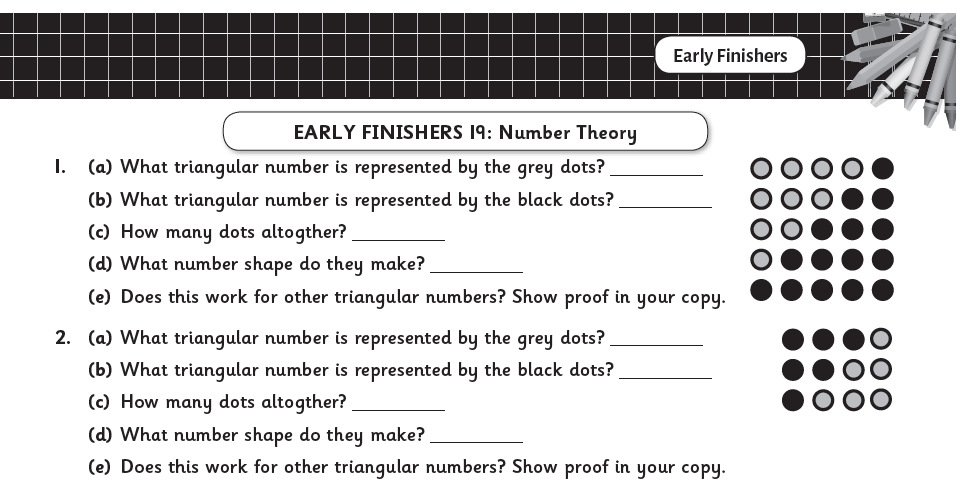### Points to note for the teacher

• Counting/Natural numbers: When we talk about numbers in this topic we are referring to the set of counting numbers (i.e. 1, 2, 3, 4 … ) or natural numbers. These are whole numbers (no fractions or decimals) and do not include negative numbers. Since there is no universal agreement about whether to include zero in the set of natural numbers (some define the natural numbers to be the positive integers {1, 2, 3, …}, while for others the term designates the non-negative integers {0, 1, 2, 3, …}) in Operation Maths we only consider the positive integers, i.e. not including zero.
• That which constitutes a rectangular number is undefined in the mathematics curriculum and the definition varies from source to source, with many conflicting with each other, particularly online. In Operation Maths, we have gone with the definition, as shown in the image below, given in the NCCA Bridging Glossary. This defines rectangular numbers as n x (n+1) which can also be expressed as n² + n; thus, the 6th rectangular number is 6 x 7 or 6² + 6 both of which equal 42.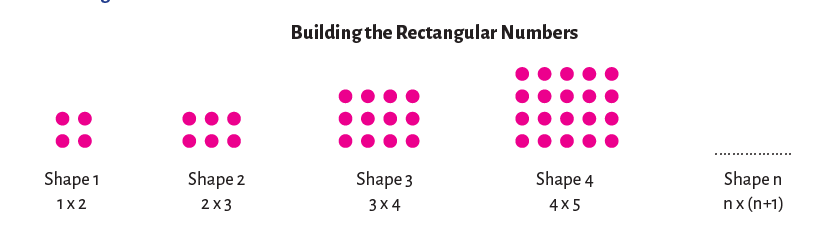• Only whole numbers can be even or odd. The children may think that 1.2 or 4.36 are even numbers since their last digits is one of 0, 2, 4, 6, 8. However, only whole numbers can be denoted even or odd; you cannot create either an even or odd cube pattern (as shown in the Operation Maths 5 Pupils’ Book, p. 153), using 1 and a bit cubes.
• Factors V multiplicands. In this sentence, 1.2 × 1.5 = 1.8, 1.2 and 1.5 are strictly speaking not factors even though they are being multiplied to produce a product. This is because factors are whole numbers, and when we ask for the factors of a particular number we want the whole number divisors of that number; if we were to include fractions (or decimal fractions) then the list would be impossibly long, complex and almost infinite. In the number sentence above, 1.2 and 1.5 are multiplicands or can also be referred to as decimal factors.
• Factor pairs should be listed in a systematic way so as to identify all the pairs, i.e. not omit any, or equally not repeat pairs. Many children forget to include 1 and its pair as a factor, or any factors that are outside the traditional limits of the 10 × 10 factors,
e.g. omitting 1 × 36, or 2 × 18 as factors of 36. The may also rewrite a pair, e.g. (2,3) and (3,2), not appreciating that because of the commutative property, order doesn’t matter and this is the same pair. To avoid these mistakes, demonstrate to the children how to identify factor pairs in a systematic way using a T-chart, as shown below.### Online Resources

• Operation Maths Digital Resources: As always don’t forget to access the linked digital activities on the digital version of the Pupil’s book, available on edcolearning.ie. Tip: look at the footer on the first page of each chapter in the pupil’s book to get a synopsis of what digital resources are available/suggested to use with that particular chapter.
• Explore exponential numbers via these lessons on the spreading of rumours and secrets.
• That Quiz: Use the factors quiz to practice identifying prime and composite numbers, prime factors, HCF and LCM. Use the exponents quiz to practice roots and exponents. To find out more about the potential of That Quiz across all strands and subjects, please read on here.
• Using Lego to develop math concepts: Read this article to discover ways to use Lego to explore square numbers, arrays and factors

• For more hints and tips specific to each class level, check out the “What to look out for” section in the introduction to this topic in the Teacher’s Resource Book (TRB)
• Number Theory Board on Pinterest

## Digging Deeper into … Multiplication and Division (3rd – 6th classes)

Category : Uncategorized

For practical suggestions for families, and links to useful digital resources, to support children learning about the topic of multiplication and division, please check out the following post: Dear Family, your Operation Maths Guide to Multiplication and Division

Children are formally introduced to the operations of multiplication and division in third class, although their initial exploration of these concepts would have begun in 1st and 2nd classes with basic skip counting in jumps of 2s, 10s, 5s and, to a lesser extent, 3s and 4s. Thus, most children become quite comfortable with the number facts of twos, fives and tens quite quickly because these facts are more familiar to them. That said, when starting multiplication and division, and particularly when doing these groups of “easier” facts, it is still very important that the children are given ample time and experiences to develop a solid understanding of the concepts of multiplication and division themselves. This is why this topic is typically taught as a double (i.e. two week) chapter in Operation Maths.

Furthermore, as with the Operation Maths approach to addition and subtraction, multiplication and division is, for the most part, taught together, so as to reinforce them as related concepts that are also the inverse of each other. Thus, the initial activities in the Discovery Book, often require the children to reflect on their understanding of the concepts and to compare and contrast them.

As the children move through the classes, it is anticipated that they would begin to use this understanding of multiplication and division as the inverse of each other, to recognise the quotient (answer) in division as a missing factor in the inverse multiplication fact, e.g. asking what is 45 ÷ 9 is the same as asking how many groups of 9 equals 45 or [ ] × 9 = 45. This knowledge will also help as the children move towards more efficient recall of the basic division facts.

### CPA Approach

As always, Operation Maths advocates a concrete–pictorial–abstract (CPA) approach to this topic. In 3rd class, this means the children will be moving from experiences with familiar objects that are already pregrouped (eg three wheels on a tricycle, four wheels on a car, ten toes on a person) and/or groups they create themselves using objects, to pictorial activities (e.g. where the children draw representations of the numbers using  pictures of the concrete materials) and finally to abstract exercises, where the focus is primarily on number sentences and the use of the multiplication and division symbols.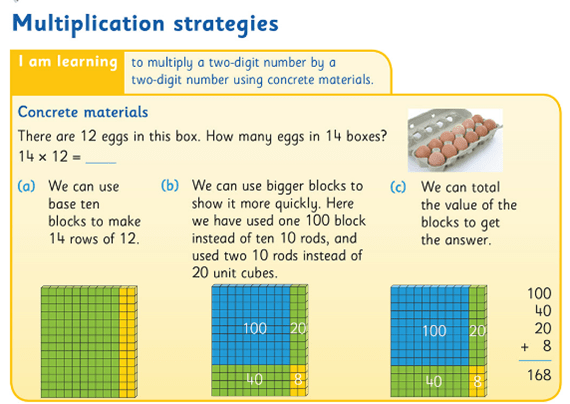Using base ten blocks to demonstrate multiplication as an area array

Similarly, in 4th to 6th classes, the approach taken should start with concrete materials (eg base-ten blocks to represent multiplication using the area model, as shown above) as demonstrated by the images in the Pupil’s books and explained in the relevant Teacher’s Resource Book (TRB). As always, the emphasis is on the children understanding the process before being introduced to the formal algorithms (abstract stage). Otherwise, they are just being taught to use a procedure to produce an answer without really understanding why or how it works.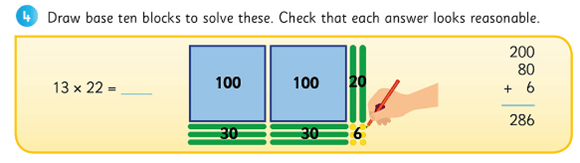Moving on from the actual blocks; drawing an area model as a pictorial representation of the calculation.

Teachers should use opportunities such as questioning, conferencing etc. to assess their children’s understanding as opposed to just checking for a correct answer.  In particular, check does the child truly understand the purpose of the zero in the second line of the long multiplication algorithm; often when questioned a child will say they need a zero or the answer will be wrong, without appreciating that they are now multiplying by a multiple of ten, so the digits must be moved up a place, hence the necessary zero as placeholder. Therefore, in the example below, 14 is first multiplied by 7, giving a product of 98 on the first line, and then 14 is multiplied by 10 (not 1) giving a product of 140 on the second line. These partial products are then added to give a final total or final product. If in doubt about a child’s understanding, return to the concrete materials and/or pictorial phase again.  Based again on the area model of multiplication, the Partial Product finder tool from the Math Learning Centre can also be very useful to demonstrate this (shown below).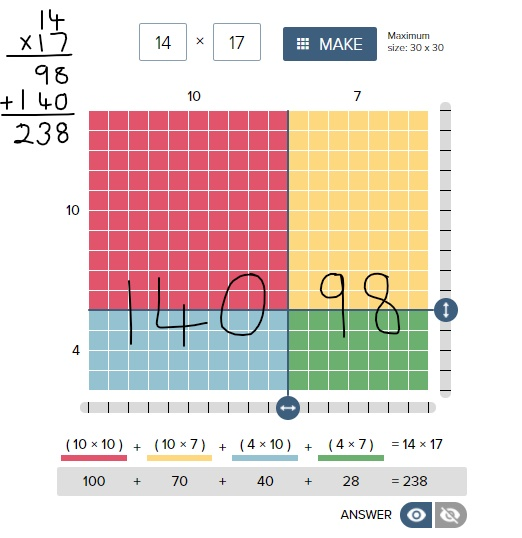If you want even more examples of the effectiveness of using the area model of multiplication, please check out this video from James Tanton. The entire facebook post is accessible here

Similarly, when considering division, while the goal is for the children to be able to divide by two-digit numbers confidently, that doesn’t necessarily have to be via the formal long division algorithm. The long division algorithm has long been a controversial element in primary mathematics. Many feel it is counter-productive to be spending so much time in 5th and 6th class learning and teaching a procedural approach to solve written calculations, when the children will have continual access to a calculator for such calculations once they hit secondary school. In fact, in many other educational systems (UK and various US states included) they have abandoned the teaching of the long division algorithm completely in preference to the ‘chunking method’ (also known as the partial quotients method). However, if the method of the long division algorithm is taught using a CPA approach, as demonstrated in the Operation Maths Pupil’s book for 5th class, it can serve to enhance children’s understanding of number and operations in general.### Language & PropertiesConsistent and accurate use of language is also an important way to emphasise the necessity of this interconnected CPA approach. The way that the number sentences for these operations are verbalised can unwittingly confuse children. If for  2 x 4, we say ‘two multiplied by four’, that implies 2 + 2 + 2 + 2, e.g. four plates with two cookies oneach. However, if we say ‘two times/groups/rows of four’, that implies 4 + 4, e.g. two plates with four cookies on each. While it is understood that the order for multiplication doesn’t matter (i.e. the commutative property of multiplication) and that the answer for both of these is the same, children don’t inherently understand this, especially at the introductory stage. And to further confuse them, when represented concretely or pictorially, both images would also look different.

Therefore, for consistency, initially teachers and students  should use  the language of ‘groups/rows of’ to describe multiplication sentences and use the word times when verbalising the multiplication symbol (×). Similarly, in division we should initially use ‘shared/divided between’ and when using the division symbol (÷) we should say ‘divided into (… equal groups)’. When comfortable with the concept of division as sharing (i.e. 12 ÷ 2 = 6, 12 divided into 2 equal groups is 6 in each group) then the children can be introduced to the concept of division as repeated subtraction (12 ÷ 2 = 6, how many groups of 2 in 12 or how many times can I take  a group of 2 away from 12).

Once the children understand that they can use the commutative property to turn around facts in order to calculate more efficiently, they should be encouraged to recognise how multiplication sentences can be distributed into smaller groups, and that smaller groups can be combined to give the same total i.e  the distributive property of multiplication. It is hoped that they will begin to recognise where these properties can be applied in order to make calculations easier. Encourage the children to read distributed number sentences using the language of ‘groups of’ or ‘rows of’, as this can aid clarity, where the numbers and symbols all together can cause confusion. The 100 dots grids on the inside back covers of Operation Maths 3 and 4 can be extremely useful for the pupils to model various arrangements/arrays, while the teacher can use the 100 square eManipulative to replicate the children’s arrangements on the IWB.Ultimately, being able to understand and apply the properties of multiplication greatly aids fact fluency. Understanding the distributive property, in particular, is key to understanding how the long multiplication algorithm works, i.e. that we multiply by the units and then multiply by the tens and then recombine these partial products. The distributive property and the ability to combine or break apart groups within factors also provides the children with strategies to mentally calculate products and quotients without having to use the standard written algorithms, for example using branching and T-charts, as featured in Operation Maths 5.

### Thinking Strategies for the Basic Number Facts

When most of us were in school, multiplication and division was largely all about tables and learning off tables. Yet, research into this area in recent years suggests strongly that memorisation and rote learning is not an effective strategy; that even when successful (which may not happen for all), the children are learning off the basic number facts, without fully understanding them, and the connections both within and between these groups of facts. Therefore, while instant recall of the basic facts (up to 10 times and divided by 10) is a primary goal of both the mathematics curriculum and of Operation Maths, Operation Maths places significant emphasis on equipping the children with thinking strategies that will enable them recall the basic facts and to compute mentally, even with numbers that are outside the traditional limits of the tables.

Some of these strategies include utilising a child’s understanding of division as the inverse of multiplication, the properties of multiplication (e.g. identity, zero, commutative and distributive) which in themselves lead into exploring connections such as doubling and halving, thirding and trebling and facts as being one set more or less another fact etc. For example, consider 9 x 5

● 9 × 5 = 5 × 9 = half 90 =45 (communtative property & 5 times as half 10 times)
● 9 × 5 = (10 x 5)- (1 x 5) = 50 – 5 = 45 (9 times as one set less than 10 times)
● 9 × 5 = treble (3 × 5) = 3 × 15 = 45 (9 times as treble, treble)
● 9 × 5 = (3 × 5) + (6 × 5) = 15 + 30 = 45 (9 times as treble plus double the treble)

That said, it would be important that these strategies are not taught purely as tricks to help arrive at an answer. Rather, the emphasis should be on the children having sufficient concrete and pictorial experiences so that they can explore and deduce the connections between groups of facts for themselves and, thus, use what they know to solve what they didn’t know.

HINT: To find out more about Thinking Strategies for Multiplication and Division, please read on here: http://operationmaths.ie/thinking-strategies-for-multiplication-and-division-number-facts/

### Mental strategies are as important as written methods

Similar to addition and subtraction, the aim is that the children will also become proficient calculating mentally, using such strategies as those outlined above, as well as using standard written methods. Once the child can use the thinking strategies to aid the recall of the basic number facts (previously referred to as tables), they can then progress to use these strategies to numbers outside of the traditional limits eg 5 x 18, 9 x 14 etc.

Again this would be very similar to the Number Talks approach to mental strategies for multiplication and division, and using the Number Talks resources accessible via the link above, alongside Operation Maths would greatly support the development of mental computation in any class.

Another important mental strategy is the ability to estimate products and quotients. Similar to the approach to Addition and subtraction, Operation Maths places particular emphasis on the development of estimation skills for multiplication and division and introduces and develops specific estimation strategies as the books progress. Once again, the emphasis should be firstly on producing quick estimates based on rounding or finding compatible numbers and secondly, on examining quickly the reasonableness of the answers e.g. number of digits. Children sometimes feel that the teacher is adding extra work by asking their students to estimate; it is important they realise that, if done quickly, estimating can greatly improve their ability to recognise errors in their own or in other’s work. However, if a child continues to have difficulties with rounding or compatible numbers, e.g. if it is taking them too much time to create an estimate, then these children should be encouraged to use front-end estimations instead, only looking at the first digit, e.g. 1,643 × 6: think 1 thousand × 6 is 6,000. While these
estimates will be less accurate than those generated by rounding, they will give the children a sense of what answer to expect in a more time-efficient way. To find out more about some of the estimation strategies, read this post.

### Problem-solving strategies

Once the children are familiar with the specific concepts of multiplication and division being taught, they should have opportunities to apply this understanding to a variety of problem solving situations, including word problems. These type of activities are typically part of the blue Work it out sections in the Pupils Books. For the children to develop their abilities to visualise the problems, they should be encouraged to use a variety of visual strategies, including bar models, branching, empty number lines, colour-coding specific operation phrases, and/or using guess and test. These various strategies are examined and developed at specific places throughout the Operation Maths books to allow the children to explore when and how they might best use them, but ultimately the child should be encouraged to use the strategy that works best for them.

In the multiplications and division chapters which are placed earlier in the school year, where the content is essentially consolidation of that covered in a previous class, some children may find the word problems quite easy to solve using a mental calculation and may be reluctant to ‘show their thinking’ in a visual/pictorial way, as they may feel that it slows them down. However, it is worth emphasising that they should get into good habits now, when the numbers involved are less challenging.

• Dear Family, your Operation Maths Guide to Multiplication & Division includes practical suggestions for supporting children, and links to a huge suite of digital resources, organised according to class level.
• Operation Maths Digital Resources: As always don’t forget to access the linked digital activities on the digital version of the Pupil’s book, available on edcolearning.ie. Tip: look at the footer on the first page of each chapter in the pupil’s book to get a synopsis of what digital resources are available/suggested to use with that particular chapter.
• For more hints and tips specific to each class level, check out the “What to look out for” section in the introduction to this topic in the Teacher’s Resource Book (TRB)
• Mental Maths handbook for Multiplication and Division from the PDST
• Number Talks book by Sherry Parrish
• Splat! Similar to Number Talks, these free resources from Steve Wyborney encourage discussion and reasoning. Play the PowerPoint presentations on your class IWB while the children use their Operation Maths MWBs to respond.
• Multiplication and Division Board on Pinterest
• Does the decimal point move? A one minute video which shows multiplying/dividing by 10/100 etc using the moving digits approach
• Can I divide by zero? A one minute video which illustrates the difficulties that arise when we consider dividing by zero
• Two more short videos from Graham Fletcher showing the progression of multiplication and division.